# Texas Go Math Grade 2 Unit 2 Answer Key Number and Operations: Computation, Money, and Equal Groups

Refer to our Texas Go Math Grade 2 Answer Key Pdf to score good marks in the exams. Test yourself by practicing the problems from Texas Go Math Grade 2 Unit 2 Answer Key Number and Operations: Computation, Money, and Equal Groups.

## Texas Go Math Grade 2 Unit 2 Answer Key Number and Operations: Computation, Money, and Equal Groups

Show What You Know

Check your understanding of important skills.

Differences to 10

Write the difference.

Question 1.
6 – 2 = _________
4
Explanation:
Difference between 6 and  2 is 4

Question 2.
7 – 4 = ___________
3
Explanation:
Difference between 7 and  4 is 3

Question 3.
10 – 4 = ___________
6
Explanation:
Difference between 10 and  4 is 6

Model Tens and Ones to 100

Write how many tens and ones. Write the number.

Question 4.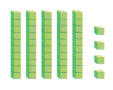__________ tens _________ ones = ___________
5 tens 4 ones = 54
Explanation:
5 tens and 4 ones is equal to 54.
50 + 4 = 54

Question 5.__________ tens _________ ones = ___________
4 tens 5 ones = 45
Explanation:
4 tens and 5 ones is equal to 45.
40+ 5 = 45

Regroup Tens as Ones.

Regroup 1 ten as 10 ones. Write how many.

Question 6.47 = 4 tens 7 ones
47 = __________ tens _________ ones
47 =  47 ones
Explanation:
1 ten = 10 ones
so
4 tens = 40 ones
47 = 4 tens 7 ones =  40 ones and 7 ones = 47 ones
Question 7.35 = 3 tens 5 ones
35 = __________ tens _________ ones
47 =  35 ones
Explanation:
1 ten = 10 ones
so
3 tens = 30ones
35= 3 tens 5 ones =  30 ones and 5 ones = 35ones

Vocabulary Builder

Visualize It
Fill in the boxes of the graphic organizer.

Review words
difference
regroup
tens
ones
digitExplanation:Understand Vocabulary

Draw a line to complete the sentence.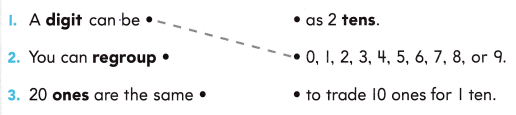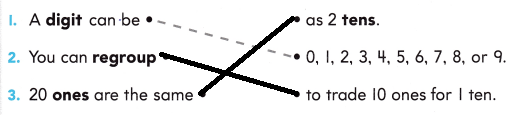Explanation:
Sentences are  matched to appropriate sentences

Tasha’s Cranes
written by Mike Mason
This Take-Home Book belongs toTasha folded paper cranes For her senior center friends. She needs 100 cranes in all. She will make them by the tens.
Explanation:
10 tens = 100 ones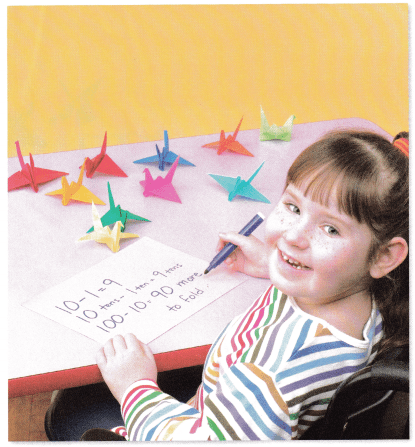Tasha started and made just 10. Then she a short rest had to take. She subtracted 10 from 100. Wow! She still had 90 more to make.
10 – 1 = 9
10 tens – 1 ten = 9 tens
100 – 10 = 90
Explanation: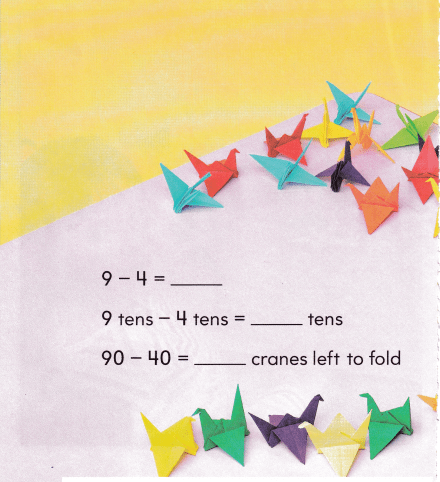The next day she folded 40 cranes. How many more does she need? Find 9 tens take away 4 tens And you will know indeed.9  – 4  = 5
9 tens – 4 tens = 5 tens
90 –  40  = 50
Explanation: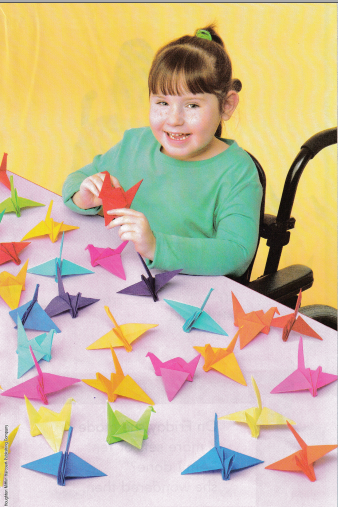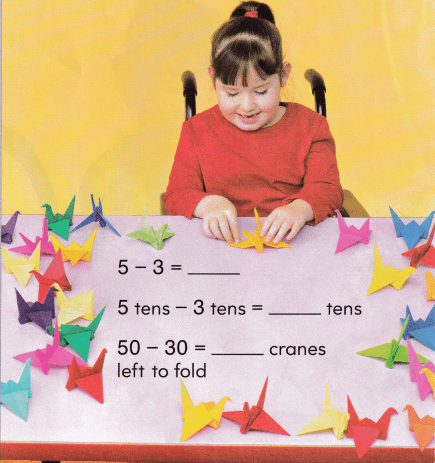On Friday she made 3 more sets of ten. “Am I done?” she wondered then.5 – 3  = 2
5 tens – 3 tens  = 2 tens
50 -30 = 20
Explanation:5 tens less 3 tens leaves 2 tens to do. So on Saturday she made 20 more. Finally 100 done. She was through!
50 + 30 + 20 = 100At the senior center she gave away the 1 100 cranes—everyone. The senior citizens thanked her for what she had done.

Write Math: Look at the picture. Draw and write your own story about the cranes. Include subtracting tens in your story.

Vocabulary Review
tens
subtract
take away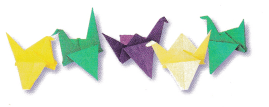Meara made 5 cranes. she counts 1 as 10.she shared 3 cranes with Meena.

How Many Tens?
5 tens – 3 tens = 2 tens

This picture shows the cranes that the children in Keisha’s class made.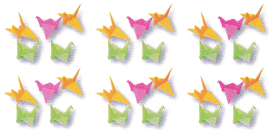Question 1.
In the picture of the cranes above, circle groups of tens. How many groups of 10 cranes are there?
__________ groups of 10 cranes
How many cranes are in the picture?
_________ cranes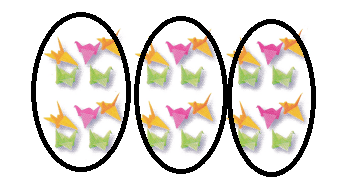3 groups of 10 cranes
3 x 10 = 30 cranes

Question 2.
Keisha’s classmates want to make 100 crones. How many tens are in 100?
_______ tens
10 x 10 = 100
there are 10 tens in the 100

Question 3.
Look at the picture of cranes above. How many more groups of 10 cranes are needed to have 100 cranes in all?
_______ more groups of 10 cranes